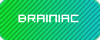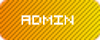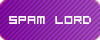# Foxide's Maths Puzzles - Introduction to Differential Calculus (*)

::  ::##Foxide's Maths Puzzles - Introduction to Differential Calculus (*)

Basic differential calculus, 1-star problems. Shouldn't be difficult - but ask around if you're having any trouble.

1) Differentiate the following with respect to x.

(i) (e^x) / cos(x)

(ii) log(x) / sin(x)

(iii)2) Differentiate the following with respect to x.

(i)(ii)(iii)3) Differentiate the following with respect to x.

(i)(ii)(iii)(iv)(v)(vi)Foxide
BrainiacNumber of posts : 180
Registration date : 2008-04-12##Re: Foxide's Maths Puzzles - Introduction to Differential Calculus (*)

I would so do these but I'm trying to do Statistics and this would only confuse meremenantNumber of posts : 451
Age : 28
Location : Essex, UK
PSN : remenant
Registration date : 2008-03-30##Re: Foxide's Maths Puzzles - Introduction to Differential Calculus (*)

Ah, statistics - that bored me quite a bit. I preferred number or group theory, or the deep, open ocean that is the Calculus. On the other hand, statisticians are wanted more so than number theorists these days!

Foxide
BrainiacNumber of posts : 180
Registration date : 2008-04-12##Re: Foxide's Maths Puzzles - Introduction to Differential Calculus (*)

true, but statistics is just formulas and learn by rote, i do kinda like the whole Core 3, 4 stuff with the exponetials and what not, exam on wednesday though, so expect an answer on thursday in the other threadremenantNumber of posts : 451
Age : 28
Location : Essex, UK
PSN : remenant
Registration date : 2008-03-30##Re: Foxide's Maths Puzzles - Introduction to Differential Calculus (*)

Please, these calculus are too easy, lets see some real math :-DGoliath
VIPNumber of posts : 98
Age : 107
MPO+ : (OHU) Goliath/ Alter-Ego
PSN : Goliath369
Registration date : 2008-06-01##Re: Foxide's Maths Puzzles - Introduction to Differential Calculus (*)

please encourage him, i'm having trouble keeping up as it is XDremenantNumber of posts : 451
Age : 28
Location : Essex, UK
PSN : remenant
Registration date : 2008-03-30##Re: Foxide's Maths Puzzles - Introduction to Differential Calculus (*)